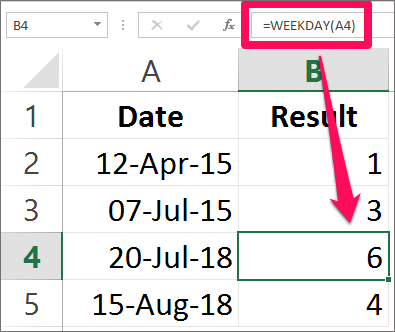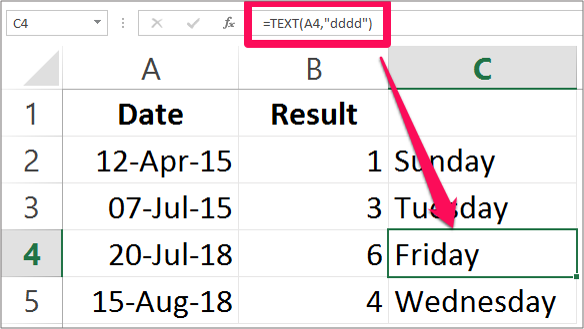# Weekday Function in Excel

## Weekday function in Excel

This tutorial will explain how you can find the day of the week in Excel by using the Weekday formula in the spreadsheet. The Weekday function returns a number which represents the day of the week of a specified date or given date. Weekday function is used for planning and scheduling purpose.

The Weekday function is an in-built function in Excel. It is located or categorized in the Date/Time function. It is used as a worksheet function and also as a VBA function in Excel.

As a worksheet function, Weekday function is entered into the cell as a formula whereas as a VBA function it is used in the Macro code using the Microsoft Visual Basic Editor.

The Weekday function returns the number between 1 and 7 which represents the day of the week. By default, the Weekday function returns 1 for Sunday and 7 for Saturday. You can use the Weekday formula along with the other formula.

This function applies to Excel 2000 and all the later versions. The Syntax for the Weekday function in Excel is:

WEEKDAY(serial_number, [return_type])

Where,

Serial number represents the date or a cell reference. It is compulsory to enter the serial number argument in this function.

Return value is optional. It determines which day of the week to be used as the first day for calculation.

Note: If the serial number and the return value in the function is numeric but out of the range specified then it will return #NUM as the value.

Let’s take a look at the example below. The image below specifies the date and the formula used along and the result of the formula.The Result Column displays the numeric value representing the day of the week. After you have found the day of the week and you want to know the name of that numeric value obtained.

That is any day from Sunday to Saturday use the Text formula as explained below. The TEXT formula here will help you to display the day of the week. The date stands for which day of the week.

The example in the image below explains you how you can use the text formula to know the day of the week. The Syntax for knowing the day of the week is:

TEXT(value, format_text)

Where,

Value represents the date. It is compulsory to enter this argument.

Text Format here is entered as “DDDD” which is the custom date format to know the day of the week.NOTE: It is advised not to type dates directly into the argument as Excel may interpret text representation of dates differently. So it advised to use cell reference instead of entering the date directly.

Hope you like our tutorial on Weekday function & Text function for more cool and amazing trick of excel Like our Excel Superstar Facebook Page and subscribe to our Excel Superstar YouTube channel. Excel Superstar is the leading online training company, which provides Online Excel Course in Hindi. Connect with us and become an Excel Superstar.